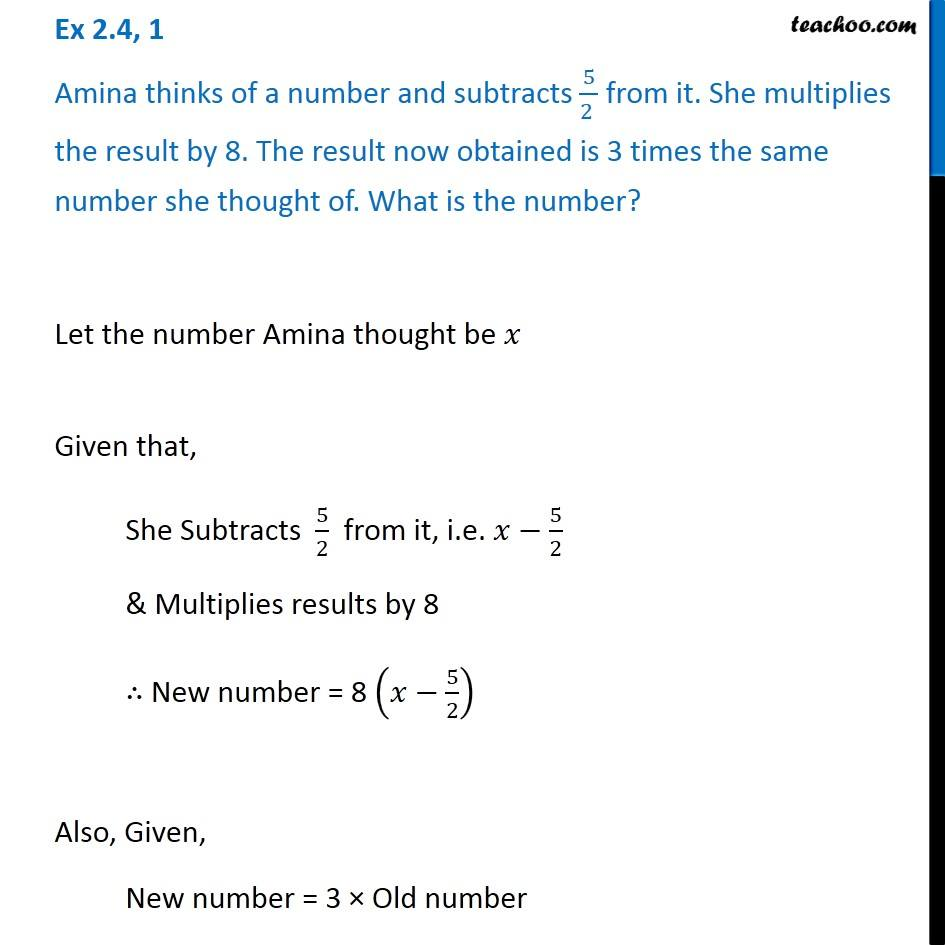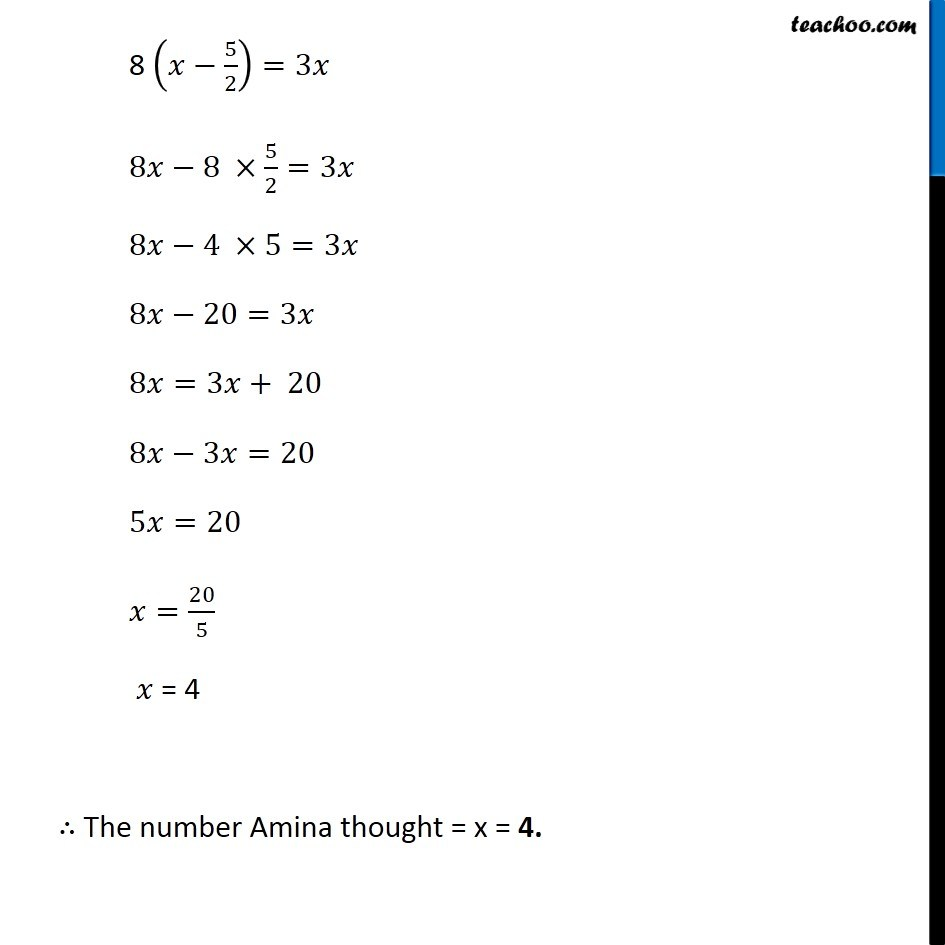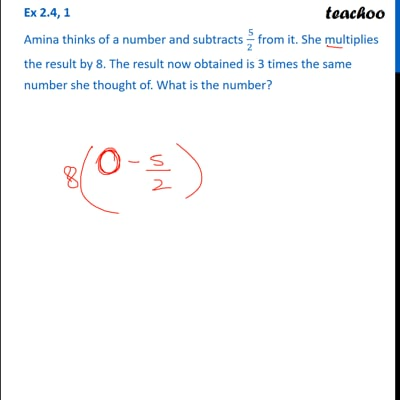Solving difficult equations

Chapter 2 Class 8 Linear Equations in One Variable
Serial order wiseThis video is only available for Teachoo black users

Learn in your speed, with individual attention - Teachoo Maths 1-on-1 Class

### Transcript

Question 1 Amina thinks of a number and subtracts 5/(2 ) from it. She multiplies the result by 8. The result now obtained is 3 times the same number she thought of. What is the number? Let the number Amina thought be 𝑥 Given that, She Subtracts 5/2 from it, i.e. 𝑥−5/2 & Multiplies results by 8 ∴ New number = 8 (𝑥−5/2) Also, Given, New number = 3 × Old number 8 (𝑥−5/2)=3𝑥 8𝑥−8 ×5/2=3𝑥 8𝑥−4 ×5=3𝑥 8𝑥−20=3𝑥 8𝑥=3𝑥+ 20 8𝑥−3𝑥=20 5𝑥=20 𝑥=20/5 𝑥 = 4 ∴ The number Amina thought = x = 4.